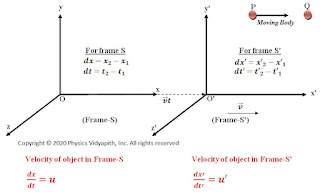### Derivation of Addition of Velocity in Special Relativity

Let us consider two frames $S$ and $S'$, frame $S'$ is moving with constant velocity $v$ relative to frame $S$ along the positive direction of the X-axis.

Let us express the velocity of the body in these frames. Suppose that body moves a distance $dx$ in time $dt$ in frame $S$ and through a distance $dx'$ in the time $dt'$ in the system $S'$ from point $P$ to $Q$. ThenAddition of velocity

$\frac{dx}{dt} = u\qquad \frac{dx'}{dt'}= u'\qquad (1)$

From Lorentz's inverse transformation

$x=\alpha\left ( x'+vt' \right )\qquad(2)$

$t=\alpha '\left ( t'+\frac{v\cdot x'}{c^{2}} \right )\qquad(3)$

Differentiate the equation $(2)$ and equation $(3)$ with respect to $t'$

$\frac{dx}{dt'}= \alpha \left ( \frac{dx'}{dt'}+v \right )\qquad(4)$

$\frac{dt}{dt'}= \alpha ' \left ({1}+\frac{v}{c^{2}} \cdot \frac{dx'}{dt'} \right )\quad (5)$

Now divide the equation $(4)$ by equation $(4)$. So

$\frac{\frac{dx}{dt'}}{\frac{dt}{dt'}} = \left ( \frac{\frac{dx'}{dt}+v}{1+\frac{v}{c^{2}}\cdot \frac{dx'}{dt'} } \right ) \qquad$

From Lorentz Transformation $\alpha =\alpha '$. So above equation can be written as:

$\frac{dx}{dt} = \frac{u' +v}{1+ \frac{u'v}{c^{2}}}$

From equation $(1)$. The above equation can be written as:

 $u = \frac{u' +v}{1+ \frac{u'v}{c^{2}}}$

This equation represents the relativistic law of addition of velocities with respect to an observer at frame-$S$ whereas in classical mechanics it is simply $u = u'+v$. There is the following point observed from the addition of the velocities equation.
1. When $u'$ and $v$ are the smaller as compare to $c$, then $\frac{v\cdot u'}{c^{2}}$ can be negligible so

 $u = u'+v$

which is classical formula.

2. When $u' = c$Then $u =\frac{c+v}{1+\frac{v}{c}}$ So,

 $u=c$

Therefore a object moves with velocity of light $c$ with respect to other, then their relative velocity is always $c$.

3. When $v=u'=c$Then $u =\frac{c+c}{1+\frac{c^{2}}{c^{2}}}=c$ So,

 $u=v$

This shows that the addition of the velocity of light simply reproduces the velocity of light. It means that the velocity of light in a vacuum is the maximum achievable velocity in nature and no signal and any object can travel faster than the velocity of light in a vacuum.